## Watanabe WVP Series Signal Converter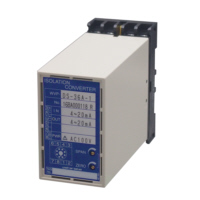Watanabe WVP Series Signal Converter

"Gọi 0974400989 để được tư vấn miễn phí"

AME

Cam kết hàng mới 100%
Bảo hành chính hãng

Watanabe WVP Series Signal Converter

・Low price.

・Easy maintenance by plug-in structure.

IsolatorsWVP-IS WVP-DS isolator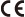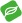isolator(Response time:25ms)isolator(Response time:200ms)isolator(Dielectric strength of 1500Vac)High-speed isolator(Dielectric strength of 2000Vac)High-speed isolator(Dielectric strength of 1500Vac)Distributors (Current Loop Supply)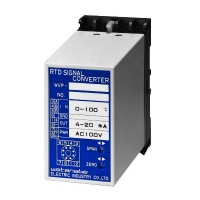WVP-DB Distributor (Current loop supply、non-isolated)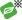Distributor(Current loop supply、non-isolated)Distributor with extraction of square root (Current loop supply、non-isolated) Distributor (Current loop supply)Distributor (Current loop supply)Temperature ConvertersWVP-KA Thermocouple temperature converter(Response time:200ms、non-isolated)Thermocouple temperature converter(Response time:200ms)Thermocouple temperature converter(Response time:25ms)RTD temperature converter(Response time:200ms、non-isolated)RTD temperature converter(Response time:200ms)RTD temperature converter(Response time:25ms)RTD temperature converter(Ni508.4Ω、Response time:25ms)Sensor Signal ConvertersWVP-DC DC converter(non-isolated)Load cell converter(non isolated)Load cell converterPotentiometer converter(Response time:200ms、non-isolated)Potentiometer converter(Response time:200ms)Potentiometer converter(Response time:25ms)Resistance to DC converter(non-isolated)Resistance to DC converterTachogenerator converter(non-isolated)Tachogenerator converterPower/AC Signal Converters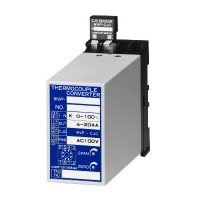WVP-CTA CT converter(Average rectified value)CT converter（true RMS）AC 2-factor converter(Average rectified value) RMS value 2-factor converter（true RMS） PT converter（Average rectified value）PT converter（true RMS）AC converter(Average rectified value、non-isolated)AC converter（Average rectified value）RMS value converter（true RMS、non-isolated）RMS value converter（true RMS）Power ConverterFrequency converter

Pulse / Frequency ConvertersWVP-FZH Pulse isolator(Dielectric strength of 2000Vac)Pulse dividerPluse rate divider(Dielectric strength of 2000Vac)Pulse isolator(Dielectric strength of 1500Vac)Pluse rate divider(Dielectric strength of 1500Vac)Pulse to DC converter(Dielectric strength of 2000Vac)Pulse to DC converter(Dielectric strength of 1500Vac)DC to pulse converter(frequency setting type、Dielectric strength of 2000Vac)DC to pulse converter(frequency setting type、Dielectric strength of 2000Vac、with shutdown function) DC to pulse converter(frequency setting type、Dielectric strength of 2000Vac、with shutdown function)2-output DC to pulse converter(frequency setting type)

Alarm Setters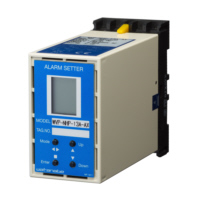WVP-NHL Alarm setter for 2-point setting(with a LCD display)Alarm setter for 1-point setting(with a LCD display)Computation ModulesWVP-RZ Square root extractor Ratio Bias Setter(Equation:X0=KXi±B、non-isolated、Response time:200ms)Ratio Bias Setter(Equation:X0=KXi±B、Response time:25ms)Ratio Bias Setter(Equation:X0=K(Xi±B)、Response time:25ms)Absolute value output converterMean Value Output ConverterAdder(non-isolated)AdderSubtractor(non-isolated)SubtractorMultiplier Divider(non-isolated) Divider

Function ModulesWVP-DBD Delay Buffer(non-isolated)Delay BufferSlope response converter(non-isolated)Slope response converterSignal limit converter (limiter)(non-isolated)Signal limit converter (limiter)Reverse converter(non-isolated)Reverse converterHigh selector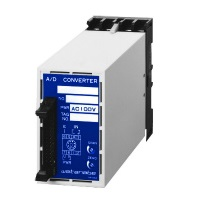WVP-ADR A/D converterD/A converterHigh-speed D/A converter